﻿ QED Lamb shift (hydrogen atom) is wrong ?

# Lamb shift is a storehouse of wrong definitions.

## QED Lamb shift is filled with a string of "mathematical" tricks.

### [ Very small value = Infinity ∞ !? ]

(Fig.1) "Infinity" must be artificially removed for Lamb shift.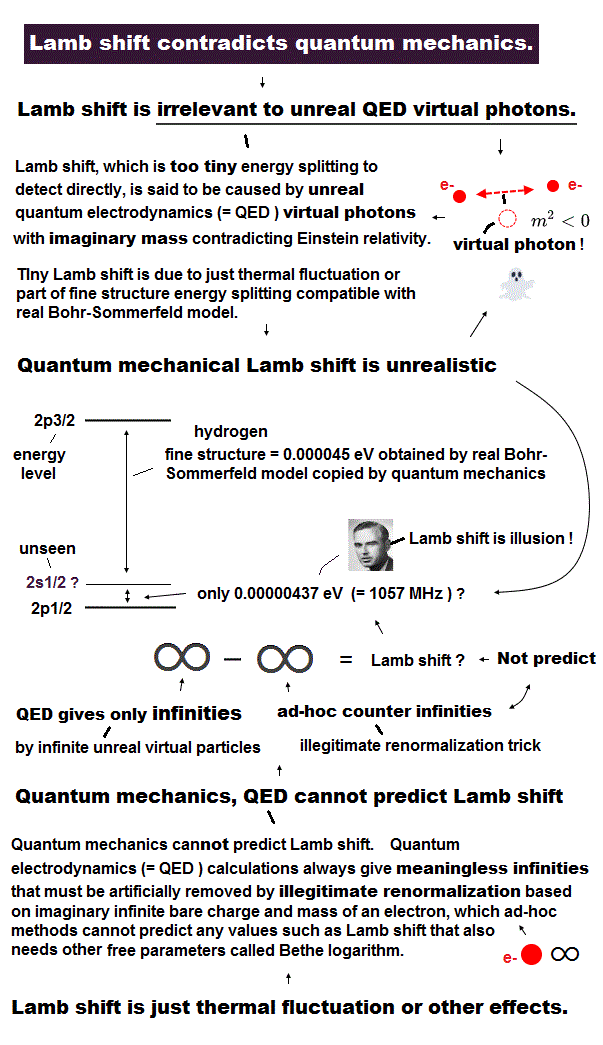According to the relativistic quantum field theory (= QFT ), the energy levels of 2s1/2 and 2p1/2 are completely the same, because they have the common j = 1/2.
As shown in this page, Lamb shift measurement is too difficult and vague in respect of accuracy.
And they rely on very speculative definition.
We cannot see what is really happening in this very small effect (= 0.0000043 eV, hyperfine level ).

Though this Lamb shift is extremely small, the calculation value becomes divergent to infinity.
This means ∞ = 0.0000043 eV. Can you believe this doubtful result ?
It is famous that Paul Dirac NEVER admitted this artificial quantum electrodynamics (QED), though Dirac equation is the heart of QED, as I explain in this page.

(Fig.2) Sommerfeld model= Dirac's hydrogen (= contains many "Wrong" states ).Surprisingly, as shown in this page, Bohr-Sommerfeld fine structure gives completely the same results as that of Dirac equation, though they are based on completely different mechanism.

Dirac equation consists of four-component spinor.
So it must contain upper and lower different-type of spinors.
For example, in the case of 2P3/2, the upper spinor means 2P3/2 state, and the lower spinor means unrealistic 2D3/2 state.
Because 2D3/2 is principal quantum number n = 2, and orbital angular momentum l = 2, which doesn't exist in Schrodinger hydrogen.

This result clearly shows Bohr-Sommerfeld fine structure is right, and Dirac equation is wrong.
And QED Lamb shift is completely dependent on this Dirac's hydrogen.
So QED Lamb shift is wrong, too.

### [ If QED g factor is wrong → Lamb shift is wrong, too. ]

(Fig.3) Lamb shift includes "wrong" vertex correction (= g factor ).As I explain later, Lamb shift includes vertex correction in it, so it uses the common results of QED g-factor.
The problem is the calculation of this g-factor (= anomalous magnetic moment ) completely depends on "wrong" math.
This means Lamb shift including the common wrong vertex correction is wrong, too.

(Fig.4) "Change of variable" ( k = l - β ) cannot change the result itself !As shown in this page, they take advantage of the change of variables in integrals, which changes the result of g-factor.
This is completely mathematical trick, and a mistake in calculation. ( Of course, intentional mistake. )
Because the change of variable is just a means, NOT influencing the results themselves, according to the "right" math.

If the first upper integral of Fig.4 is zero, the second integral (= middle ) becomes zero, because they are the same integral.
( Only change of variables is done in the second integral. )
But the same integrals give different results ( one is zero, another is not zero ) in the lowest integral in Fig.4.
This is completely wrong math, and intentional trick.

### [ Many different terms must be "artificially" combined to get Lamb shift = Mathematical trick. ]

(Fig.5) Tiny Lamb shift is "packed" with many different effects !? = "ad hoc" mathematical trick.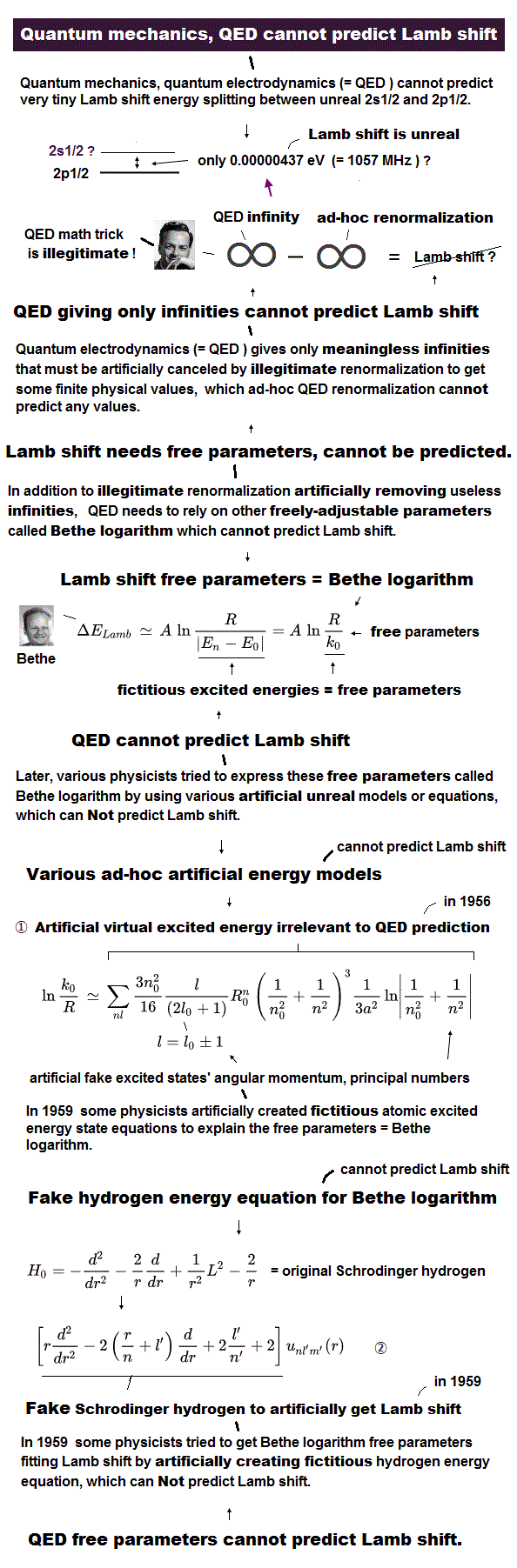Anomalous magnetic moment consists only of one vertex correction of Fig.3.
On the other hand, QED Lamb shift is much more complicated and filled with artificial tricks.

First you need to divide relativistic high energy and nonrelativistic low energy with respect to virtual photon (= k ).
Furthermore, self-energy of virtual photon (= vacuum polarization of Fig.5 middle ) needs to be incorporated into it to be close to experimental value. These parts correspond to Dirac's hydrogen.
Finally, the perturbation energy in Schrodinger equation must be computed. ( Very crowded inside extremely tiny value ! )

As shown in this page, the lower spinor of Dirac's hydrogen is a fictional and wrong state.
But in QED Lamb shift, they combine lower and upper spinors of Dirac hydrogen, and then combine them with Schrodinger's wavefunction of low virtual energy. So they treat these virtual spinors as "real" state.
This is clearly unreasonable, and proves QED Lamb shift is wrong.

## Unrealistic excited energies are the most important trick.

(Fig.6) 2S is excited to 226.4 eV, on the other hand, 2p is only 13.2 eV !?The most important tricks exist in virtual (= random ) excitation energies (= Bethe logarithm ).
They introduced this strange concept without offering any reasons.

According to the Quantum theory of Fields ( S. Weinberg ), the random excited energies from 2s is 226.4 eV. See this site (p.6).
On the other hand, the excited energy from 2p state is vary small (= 13.2 eV ).

This wide discrepancy is also one of the main causes of Lamb shift.
Unfortunately, there were NO proper reasons about these unrealsitic, virtual excitation energies.

It is very unnatural and impossible that each electron is always excited to high energy state (= 226.4 eV ) within very small Lamb shift interval (= 0.0000043 eV ) !

And these random excited energies have to rely on "numerical" methods.
So, "precise" calculation of Lamb shift is very doubtful.

## Simple calculation of QED Lamb shift.

### [ QED Lamb shift depends on "wrong" math ! ]

(Fig.7) Wrong approximation of Dirac's hydrogen.To get Lamb shift, we have to use wavefunction such as 2S1/2.
But QED uses relativistic Dirac equation, which originally doesn't have concept of wavefunction.
So they need to change Dirac's hydrogen eigenstates into real nonrelativistic wavefunction by force.
In this approximation, they use completely wrong math (= Fig.7 ), as I explain in this section.

(Eq.1) Dirac equation for hydrogen atom interacting with electromagnetic fields (= A, V ).As shown in this page, Eq.1 means Dirac equation for hydrogen atom.
Eq.1 includes the interaction with electromagnetic fields ( see this page ).
"A" is vector ( magnetic ) potential, and "V" is scalar (electric) potential.

σ is Pauli matrices.
Of course, ψ is four component spinor (= 4 × 1 spinor ).

(Eq.2)We separate mass energy (= mc2 ) from other spinor parts, as shown in Eq.2.
So, φ and χ contains only kinetic energy, they insist.
These φ and χ are two component (= 2 × 1 ) spinors.
But in fact, their insistence is completely wrong in the lower spinor χ.

Substituting Eq.2 into Eq.1,
(Eq.3)From Eq.3, the upper and lower equations become

(Eq.4)As shown in Eq.4, mass energy term (= mc2 ) is cancelled out in the upper equation, due to separation of mass energy.
But ( negative ) mass term of lower equation becomes twice the original value.
So in the lower equation, this (negative) mass energy becomes much bigger than the original form, which cannot be neglected.

(Eq.5)Here electromagnetic field is approximately considered to be zero.

(Eq.6)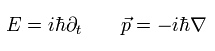In quantum mechanics, time and space derivatives mean energy (= E ) and momentum (= p ), respectively.
Using Eq.5 and Eq.6, Eq.4 appoximately becomes

(Eq.7)As shown in Eq.7, the energy of the lower equation becomes too big due to 2mc2 negative energy, which cannot be neglected.
( So as I said, the lower spinor of Dirac equation isn't real state. )
But they artificially treat this energy value (= time derivative ) as zero for getting Lamb shift.

(Eq.8) Left side is zero ? ← trick.In the lower equation of Eq.4 ( see also Eq.8 ), they artificially delete energy term of left side (= time derivative ).
Because this energy term doesn't contain the light speed "c" at all, they insist.
But this interpretation is completely wrong.
The light speed "c" is tacitly included in eneregy itself as ( E = cp ).

If Eq.8 is satisfied, the energy term (= time derivative ) of left side becomes as big as -2mc2, which cannot be neglected, and cannot be zero.
But they deleted left side of Eq.5 as zero. This is strange.
This mathematical artificial trick is introduced only to obtain experimental value of Lamn shift.

### [ Unrealistic virtual photon is more important in Lamb shift. ]

(Eq.9) Virtual photon disobeys special relativity.As shown in this page, we condsider one electron (= p ) absorbs ( or emits ) photon (=q ), changing its momentum p into p' (= final momentum of electron ).
In this case, when the energy and momentum are conserved between the electron and photon, this photon "q" always contradicts special relativity.
( This photon q always becomes "tachyon" in Einstein mass energy relation. )

So this photon q is called "virtual photon".
Here ( -1, 1, 1, 1 ) version of metric tensor is used. See this page.

(Eq.10) One loop vertex correction → divergent to infinity.Lamb shift includes one loop vertex correction of anomalous magnetic moment (= g-factor ) as a part of it.
So if QED g-factor calculation is wrong, of course, QED Lamb shift is wrong, too.

Here we consider Eq.10 case, in which "q", "k" and "p-k" are virtual photon, photon propagator, electron propagator, respectively.

### [ Virtual photon q = 0 → g-factor ( anomalous magnetic moment ) is obtained. ]

(Eq.11) Unrealistic virtual photon "q" needs to be zero for getting g-factor.To get g-factor (= anomalous magnetic moment ), we have to use the condition of q2 = 0 (= Einstein mass relation ).
This photon q is "virtual" tachyon, so if this realistic condition is satisfied, all energy and momentum components of "q" become zero, which means virtual photon disappears.

In Eq.11, "α" means fine structure constant.

### [ Virtual photon needs to be artificially "revived" for getting Lamb shift. ]

(Eq.12) Virtual photon q2 = 0 or Not zero ?   Which is true ?The important point is that we have to artificially revive this unrealistic virtual photon term (= q2 ) for getting Lamb shift.
F1 term of Eq.12 is virtual photon term.

On the other hand, Lamb shift also needs g-factor term, in which q2 is zero.
So QED Lamb shift depends on two contradictory terms of q2 = 0 and NOT zero.
This is clearly self-contradiction, and shows QED Lamb shift is the result of artificial mathematical tricks.

### [ Dividing high energy and low energy terms ? ]

(Eq.13) High energy terms.To avoid infrared divergence, they artificially separate high energy and low energy terms.
Eq.13 means high energy terms with respect to virtual photon "k".

The first term of the right side of Eq.13 is one loop vertex correction of Eq.12.
And the second term of the right side is low ( relativistic ) energy term, which becomes

(Eq.14) Low ( relativistic ) energy terms in vertex correction.As I said above, this q2 is unrealistic virtual photon term.
But they consider this unrealistic term as real value.

### [ Different vacuum polarization is needed for Lamb shift. ← mathematical trick. ]

(Eq.15) Vacuum polarization, photon self energy term is also needed ?To be close to the experimental value of Lamb shift, another type of self energy is indispensable. (= intentional definition ! )
Eq.15 is virtual photon self energy, which becomes divergent to infinity.
Only finite term is used as a part of Lamb shift.

So they try to intentionally combine various different kinds of effects to get extremely tiny Lamb shift.
You find that QED Lamb shift is NOT a natural result of quantum electrodynamics.

Substituting Eq.12, Eq.14 and Eq.15 into Eq.13, we get

(Eq.16) High energy term (= relativistic Dirac term ).Here we use the wrong replacement of
(Eq.17)As shown in Eq.17, q2 originally does NOT mean a square of momentum, but means photon mass term (= almost zero ).
But they replace this (virtual) mass term q2 into momentum ∇2.
This is completely wrong math, because this photon has zero energy ( q0 = 0 ), but its momentum q is NOT zero.
( This is very strange particle ! )
They have to rely on this wrong math for getting Lamb shift.

(Eq.18) Spin = Dirac matrices γThey replace spin part of σ using γ matrices, as shown in Eq.18.
And the interaction Hamiltonian between electromagnetic field and electron at vertex is
(Eq.19)In Eq.19, they suppose magnetic field Ai ( i = 1, 2, 3 ) is zero, and only A0 is not zero.
But this definition is inconsistent, because, they need vector potencial correction (= Ai ) for getting Lamb shift, as I explain later.

Using Eq.17, Eq.18 and Eq.19 in Eq.16, we get high energy term of
(Eq.20)Here, ( c=1, ħ = 1 ) is used.
As shown in these calculations and articial definitions ( replacement ), we cannot say Eq.20 is a natural result of QED.

### [ Dirac's hydrogen can be unified into Schrodinger's hydrogen naturally ? ← Impossible. ]

(Eq.21) Wrong approximation.To get Lamb shift between 2S1/2 and 2P1/2, we have to treat Dirac's four component spinor as Schrodinger's hydrogen.
But as I said in this page, lower two components of Dirac spinor are NOT real states.
Furthermore, they need to use wrong approximation of Eq.21 (= Eq.8 ) to get Lamb shift.

Using Eq.21, the first term of Eq.20 becomes

(Eq.22)where
(Eq.23)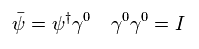As I said in Eq.21, this ψ (= four component spinor ) consists of upper (= φ ) and lower (= χ ) spinors.
But they use ONLY upper two spinor (= φ ) as this ψ, which is completely wrong math.
( 4 × 1 spinor = 2 × 1 spinor !? )
They insist this replacement is only nonrelativistic approximation. But completely unreasonable approximation.

(Eq.24) "Wrong" replacement.   Four component spinor → two component spinor !?In Eq.24, the original ψ is 4 × 1 spinor, and φ is 2 × 1 (upper) spinor.
The second term of Eq.20 is

(Eq.25)Though they artificially change four component spinor ψ into two component spinor φ in the first term, as shown in Eq.24, they try to use both upper and lower spinors only in the second term (= Eq.25 ).
Because Eq.25 includes γ matrices, which mix upper and lower spinors.
But if they use this four component Dirac spinor in Eq.25, they must use this spinor also in Eq.24.
( This is clearly self-contradiction. )

(Eq.26) Four component spinor → four component spinor.Substituting wrong approximation of Eq.21 into Eq.26, and we use the following formula,
(Eq.27)We get
(Eq.28)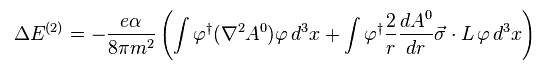### [ Low energy term = Schrodinger ( or Bethe ) equation. ]

(Eq.29) Schrodinger equation interacting with electromagnetic fields.In the upper section, we compute high energy term of Dirac hydrogen, in which Dirac hydrogen of low energy is removed, as shown in Eq.13.
To fill this vacancy, they use Schrodinger equation as low energy term.
( This manipulation is to avoid infrared divergence, they insist. But they introduced various wrong definitions in this process "secretly" ! )

(Eq.30) Only magnetic potential is not zero ?When they consider interaction wirh electromagnetic field, they suppose only magnetic potential is not zero ( electric potenial A0 is zero ), as shown in Eq.30.

As I explain in this page, the second perturbation of this interaction Hamiltonian becomes

(Eq.31)In Eq.31, ( m, k, ε ) means all middle states of virtual photons.
And "n" means the hydrogen electron's eigenstates of
(Eq.32)Vector potential A is
(Eq.33)And the electron's velocity "v" ( I don't understand why velocity "v" appears in nonlocal Schrodinger's equation ), and photon polarization (= ε ) satisfies
(Eq.34)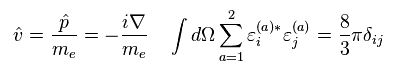Using Eq.31, Eq.33 and Eq.34, the low energy term of Schrodinger's hydrogen becomes

(Eq.35)Though they define electric potential (= A0 ) is zero (= Eq.30 ), they revive this electric potential in the final form of Eq.35.

### [ Summary of artificial trick in unifying Dirac and Schrodinger potentials Aμ. ]

(Fig.35') Mathematical trick.They insist they can get the common second derivative (= 2A0 ) of electric potential A0 in Dirac (= Eq.20 ) and Schrodinger (= Eq.35 ) equations.
But as you see this calculation process, these are completely artificial mathematical tricks.

As shown in Eq.16 and Eq.17, second derivative of Dirac part originates in unrealistic virtual photon "q".
In this virtual term, they change the definition of q2 = 0 ( ← Einstein mass formula ) into momentum derivative (= ∇2 ), which is completely wrong and ad-hoc manipulation !

On the other hand, in Schrodinger's part, they suppose magnetic potential "Ai" is not zero, and electric potential A0 is zero.
So the second derivative in Schrodinger's part originates in magnetic ( Not electric ! ) potential of Eq.29 and Eq.30.
But in the final form, they suddenly change the definition, in which only electric potential A0 is not zero.

### [ Very tiny value of Lamb shift is the result of various contradictory effects. ]

(Eq.36) Tiny Lamb shift is very crowded with various complicated effects ??Summing Eq.24, Eq.28 and Eq.35, they obtain the Lamb shift of Eq.36.
Eq.36 spin-orbital term is
(Eq.37)And you need to rely on approximate numerical calculation in the terms including velocity "v".
( So this part is vague and doubtful. )
So, the calculation result becomes
(Eq.38)This calculation value of Lamb shift is close to the experimental value of 1057 MHz.
So they insist this QED method is most successful one in the history.
But as you see in this page, they use various wrong math and artificial definitions to get Lamb shift.

They replace spin-orbital term like Eq.37.
But this replacement is impossible in Dirac hydrogen.
Because the upper and lower spinors of Dirac hydrogen have different orbital angular momentum (= l )
( Only the total angular momentum j is the common value. )

(Eq.39) Uniting Dirac's hydrogen into Schrodinger's is impossible.So they cannot determine a single orbital angular momentum in calculating 2S1/2 or 2P1/2 energy.
Again, this final definition is wrong.

## They just "artificially" distinguish 2s and 2p.

(Eq.40) Distinguishing 2s and 2p = mathematical trick ?As shown on this site, the energy gap between 2s1/2 and 2p1/2 levels in hydrogen is said to be only 0.00000437 eV (= Lamb shift ).

Physicists involved in quantum electrodynamics (= QED ) claim that this tiny Lamb shift proved the validity of QED.
But unfortunately, Lamb shift by QED method is filled with artificial mathematical tricks.

Physicists at that time tried to find a "convenient" way to distinguish 2s and 2p energy levels.
To do so, they had to rely on unphysical methods, which means QED is NOT correct theory at all.

## They utilized the derivative of potential energy for distinguishing them.

(Eq.41) Derivative of potential energy (= A0 )It is known that the derivative of Coulomb potential energy (= A0 ) gives electric field (= E ).
Furthermore, the divergence of electric field is equal to the charge density (= ρ ) divided by the permittivity.

(Eq.42) Divergence of electric field (= E ) gives charge density (= ρ ).In hydrogen-like atoms, the nuclear charge (= Ze ) is supposed to exist only at the origin (= 0, 0, 0 ), as a point particle.
So the charge density can be expressed using delta function (= δ(x) ).

## Delta function   →   "distinguish" 2s and 2p ?

(Eq.43) Delta function = convenient tool.Delta function always becomes zero except for the origin. This property can be utilized to distinguish 2s and 2p states, because only the probability of 2s at the origin is not zero.

## They must get delta function by all means = Trick.

(Eq.44) Trick = getting delta function (= δ ) by "unreasonable" methods.If they can get this delta function, the 2s and 2p states can be distinguished, they think.
Because the probability at the origin in Schrodinger's hydrogen is not zero only in 2s.

So physicists tried to get this delta function, even if its method is unreasonable and inconsistent from the realistic viewpoint.
This is the most important trick of QED Lamb shift.

## But the probability "densities" are "zero" Both in 2s and 2p.

(Eq.45) In 2s and 2p, the probability density at the origin is zero !The probability density is given by the probability amplitude × r (= radius ).
So, in fact, the probability density at the origin becomes zero also in 2s.

But if they admit this fact as it is, they cannot get Lamb shift by QED !
This is one of the reasons why these methods are incorrect also from the viewpoint of physics.

## Virtual photons contradicting relativity are indispensable.

(Eq.46) They intentionally revived the parts of virtual photons (= q ).The vertex correction is a part of complicated Lamb shift.
As shown on this page, the variable "q" means virtual photon, which violates special relativity like tachyon.

So in the last step, this q2 must be zero ( or renormalized ) to get some physical (= realistic ) values.
But to get Lamb shift, they must revive and utilize this "unreal" virtual photons.

## Virtual photon q = tachyon !?

(Eq.47) Virtual photon disobeys special relativity.As shown in Eq.47, this virtual photon is NOT a real particle.
Its total energy (= q0 ) is zero, but its momentum is NOT zero !   This is impossible.

## They pick up "convenient" terms from this virtual photons.

(Eq.48) Artificial choice. ↓To get Lamb shift, they had to pick up Only convenient terms included in the virtual photon terms (= q2 ).
This is clearly artificial choice, and means this method is NOT first principle.

In Eq.48, μ is virtual photon's mass.
So this term becomes divergent to infinity when μ returns to zero.

## Virtual photon (= q ) changes into differential operator (= ∇ ) !?

(Eq.49) Unreal virtual photons (= q ) can be used to get "real" values ?Surprisingly, they suddenly transform this "unreal" virtual photon (= q ) into the differential operator (= ∇ ).
As I said in Eq.42, the second derivative of potential (= A0 ) gives delta function (= δ ).

If this delta function can be gotten, they can distinguish 2s from 2p, at the origin, they insist.

(Eq.50) Lamb shift is a "storehouse" of artificial tricks.They tried to utilize various effects such as vacuum poralization and radiation to be close to experimental values.
All these terms contain unreal virtual photons (= q ).

The square of this virtual photon's momentum changes into the second derivative of potential. ( q2 → ∇2 ! )
Depending on this unreal virtual photon means these values cannot be considered "real".

## Another artificial method for getting the second derivative (= ∇2 ).

(Eq.51) Electron's momentum operator (= iħ∇ ) can be used as the derivative of photon !?In low energy photon term (= nonrelativistic Schrodinger's terms ), they utilized the electron momentum operator to get the second derivative of potetnial.

In Eq.51, "A" means vector potentials, which describe classical Lorentz force.
They picked up only "A∇" term as interaction Hamiltonian.

(Eq.52) Second perturbation of this interaction Hamiltonian (= H' ).Eq.52 shows the second perturbation energy based on this interaction Hamiltonian.
Later, we add the potential (= A0 ) to this equation, as a result, the second derivative can be gotten like Eq.50.

Non relativistic terms were gotten by Bethe, first

## Virtual photon and electron's momentum can be combined ?

(Eq.54) Two mechanisms are completely different, one is real and another is unreal.Comparing Eq.50 (= virtual photon ) and Eq.52 (= electron momentum ), you would think this method is inconsistent and unreasonable.
In Eq.50, they get the second derivative from unreal virtual photons (= q ).

On the other hand, in Eq.52, they use realistic electron's momentum operator to reach this derivative.
Surprisingly, they add up all these terms based on completely different concepts to get Lamb shift !

## "Artificial" excited energies are indispensable to get Lamb shift ?

(Eq.55) Two virtual excited states.These nonrelativistic terms include two different kinds of excited states (= of course, "virtual excitation" ).
"Em" is excited electron's energy in hydrogen atom.

"E" is excited energy of virtual photon (= vector potential A ).
Of course, these are just artificial definitions, and NOT first principle.Here they have to add excessive term to get Lamb shift.
Some books say this can be done using mass renormalization, other books say this term expresses vacuum photons.

But unfortunately, there are NO consistent reasons in this manipulation.
This trick is just for getting second derivative of potential , as I said above.

The Quantum theory of field ( S. Weinberg ) tries to justify artificial mass renormalization.
They try to change the interpretation of infinitely heavy mass (= Λ → ∞, "μ" in this book ) of Pauli-Villars regularization.
Of course, this changing ( Λ → 0 ? ) contradicts the original definition by Pauli-Villars.

(Eq.57) Second derivative of potential.The defferential operator (= ∇ ) originates from electron's momentum (= de Broglie wavelength ).
But they change this original meaning, as they like. ( electron → photon. )

(Eq.58) Another trick.Furthermore, they artificially delete various obstructive terms under the name of "approximation".
These are also important tricks in Lamb shift.

## Unrealistic excited energies are the most important trick.

(Eq.59) 2S is excited to 226.4 eV, on the other hand, 2p is only 13.2 eV !?The most important tricks exist in virtual (= random ) excitation energies (= Bethe logarithm ).
They introduced this strange concept without offering any reasons.

According to the Quantum theory of Fields ( S. Weinberg ), the random excited energies from 2s is 226.4 eV. See this site (p.6).
On the other hand, the excited energy from 2p state is very small (= 13.2 eV ).

This wide discrepancy is very strange, and one of the main causes of QED Lamb shift.
Unfortunately, there were NO proper reasons about these unrealsitic, virtual excitation energies.

It is very unnatural and impossible that each electron is always excited to high energy state (= 226.4 eV ) within very small Lamb shift interval (= 0.0000043 eV ) !

And these random excited energies have to rely on "numerical" methods.
So, "precise" calculation of Lamb shift is very doubtful.2014/9/26 updated. Feel free to link to this site.